# JP4771998B2 - Electric motor drive - Google Patents

## Info

Publication number
JP4771998B2
JP4771998B2 JP2007174518A JP2007174518A JP4771998B2 JP 4771998 B2 JP4771998 B2 JP 4771998B2 JP 2007174518 A JP2007174518 A JP 2007174518A JP 2007174518 A JP2007174518 A JP 2007174518A JP 4771998 B2 JP4771998 B2 JP 4771998B2
Authority
JP
Japan
Prior art keywords
motor
calculation
detection
voltage
axis
Prior art date
Legal status (The legal status is an assumption and is not a legal conclusion. Google has not performed a legal analysis and makes no representation as to the accuracy of the status listed.)
Active
Application number
JP2007174518A
Other languages
Japanese (ja)
Other versions
JP2008092787A (en
Inventor

Original Assignee

Priority date (The priority date is an assumption and is not a legal conclusion. Google has not performed a legal analysis and makes no representation as to the accuracy of the date listed.)
Filing date
Publication date
Priority to JP2006239895 priority Critical
Priority to JP2006239895 priority
Application filed by 三菱電機株式会社 filed Critical 三菱電機株式会社
Priority to JP2007174518A priority patent/JP4771998B2/en
Publication of JP2008092787A publication Critical patent/JP2008092787A/en
Application granted granted Critical
Publication of JP4771998B2 publication Critical patent/JP4771998B2/en
Active legal-status Critical Current
Anticipated expiration legal-status Critical

• 244000171263 Ribes grossularia Species 0.000 claims description 81
• 230000004907 flux Effects 0.000 claims description 60
• 239000002529 flux Substances 0.000 claims description 60
• 238000006243 chemical reactions Methods 0.000 claims description 34
• 230000001360 synchronised Effects 0.000 claims description 18
• 230000000875 corresponding Effects 0.000 claims description 3
• 230000001276 controlling effects Effects 0.000 claims 2
• 230000014509 gene expression Effects 0.000 description 15
• 230000000051 modifying Effects 0.000 description 12
• 238000010586 diagrams Methods 0.000 description 8
• 230000007274 generation of a signal involved in cell-cell signaling Effects 0.000 description 8
• 230000001965 increased Effects 0.000 description 7
• 238000000034 methods Methods 0.000 description 6
• 239000003570 air Substances 0.000 description 4
• 230000005284 excitation Effects 0.000 description 4
• 238000004519 manufacturing process Methods 0.000 description 4
• 239000011901 water Substances 0.000 description 3
• 239000000969 carriers Substances 0.000 description 2
• 239000002131 composite materials Substances 0.000 description 2
• 230000000694 effects Effects 0.000 description 2
• 238000001914 filtration Methods 0.000 description 2
• 230000001939 inductive effects Effects 0.000 description 2
• 238000005406 washing Methods 0.000 description 2
• 238000001514 detection method Methods 0.000 description 1
• 238000004870 electrical engineering Methods 0.000 description 1
• 230000001105 regulatory Effects 0.000 description 1
• 238000005070 sampling Methods 0.000 description 1
• 238000009423 ventilation Methods 0.000 description 1

## Images

•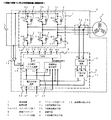•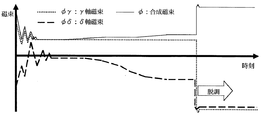•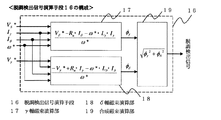•••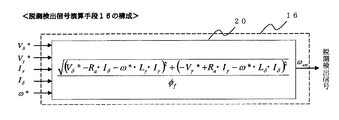•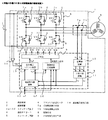•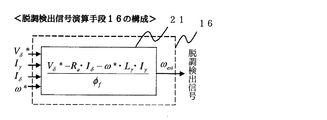••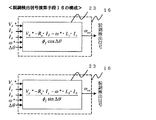•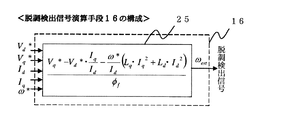•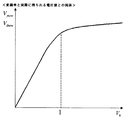••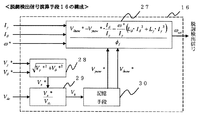•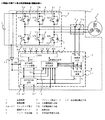••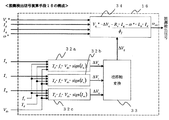•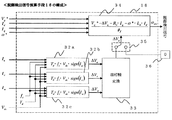•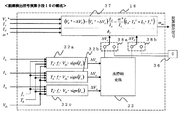## Description

The present invention relates to an apparatus for driving an electric motor.

Conventionally, in a synchronous motor drive device, as a technique for detecting a step out of a synchronous motor, “... time k · Ts (where k = 0, 1, 2, 3,..., Ts is a sampling time) By detecting the stator current for at least two phases supplied to the synchronous motor and converting the stator current into the γ-δ coordinate system, the γ-axis current iγ (k) and the δ-axis current iδ (k) are derived. The difference iγ (k) − between these γ-axis current iγ (k) and δ-axis current δ (k) and the γ-axis current iγest (k) and δ-axis current iδest (k) estimated in the previous control loop. iγest (k) and iδ (k) −iδest (k) are correction amounts, and voltage command values Vγ * (k) and Vδ * (k) converted to the γ-δ axis coordinate system are input, and the rotation of the synchronous motor Γ-axis induced voltage εγ (k) and δ-axis induced voltage εδ ( k) as a disturbance to the current response when the rotor is not rotating, and the state estimator is constructed, and the currents iγest (k + 1) and iδest (k + 1) in the γ-δ axis coordinate system of time (k + 1) · Ts seconds And the induced voltages εγest (k + 1) and εδest (k + 1) are estimated, and the step-out is detected from the estimated induced voltages εγest (k + 1) and εδest (k + 1). Detection method. (Patent Document 1).

“The voltage / frequency calculating circuit 34 of the driving device 37 calculates the operating frequency and operating voltage based on the frequency command value F *, and performs switching control and PWM control of the inverter circuit 13 via the gate driving circuit 35. The sensorless brushless motor 29 is driven. The current detection circuit 39 detects the current of the motor 29 via the current sensors 31 to 32, and the current cycle detection circuit 40 detects the cycle of the motor current. The voltage / frequency calculation circuit 34 calculates the cycle of the voltage applied to the motor 29 based on the operating frequency, and the cycle comparison circuit 41 compares the cycle of the motor voltage with the cycle of the motor current. When they do not match, it is determined that the step-out has occurred and a high-level output signal is supplied to the voltage / frequency arithmetic circuit 34. In a sensorless brushless motor driving apparatus that is operated without detecting the magnetic pole position of a permanent magnet rotor by a magnetic pole position sensor, and current detection means for detecting the current flowing through the sensorless brushless motor, A dq coordinate conversion means for converting the current signal obtained from the current detection means into an excitation current component and a torque current component, and an excitation current component obtained from the dq coordinate conversion means and an arbitrarily set step-out level signal. A step-out detecting device for a sensorless brushless motor, comprising a step-out level comparison means for comparing and determining step-out. Is also known (Patent Document 2).

Furthermore, in the operation control apparatus that supplies driving power to the DC brushless motor M that does not have a rotation detection sensor by the power switching circuit 11, a step-out detection that detects that the operation state of the DC brushless motor is in a step-out state. Means 15 were provided. This step-out detection means can be used as a means for detecting a change in the amount of water supplied or the pressure of water supplied by, for example, a pump connected to a DC brassless motor. Further, step-out detection may be performed from a change in the rotational speed of the motor or a change in the power factor. Is also known (Patent Document 3).

In addition, there is a document disclosing a method for estimating an axis error in sensorless control of a synchronous motor (Non-Patent Document 1).

Japanese Patent Laid-Open No. 11-18499 (Claim 2) JP 2001-25282 (Abstract, Claim 2) JP 2002-58281 A (summary) Electrical Engineering D, Vol.110, No.11, p. 1193-P. 1200, 1990

However, since the conventional technique disclosed in Patent Document 1 requires means for estimating the induced voltage of the motor for step-out detection, the processing load on the microcomputer or the like is increased.
Further, in the prior art disclosed in Patent Document 2, the step of detecting the step-out by comparing the cycle of the voltage output from the inverter device with the cycle of the current flowing through the sensorless brushless motor. In a state where there is no difference between the output voltage cycle and the cycle of the current flowing through the synchronous motor, step-out detection is impossible.
Also, in the method of detecting step-out by comparing the d-axis current that is the excitation current component with the step-out detection level, the step-out detection level is operated because the excitation current changes depending on the conditions of the rotation speed and load torque. It is necessary to set for each condition, and the setting becomes complicated.
Further, in the prior art disclosed in Patent Document 3, the rotational speed of the rotor is not estimated based on the operation theory of the synchronous motor, but the position information of the induced voltage is detected by a sensor or the like. A lot of manufacturing costs are required.

The present invention has been made to solve the above-described problems, and an object of the present invention is to provide an electric motor drive device capable of accurately detecting step-out of an electric motor based on the operation theory of the electric motor. It is what.

An electric motor drive device according to the present invention is an electric motor having an inverter that applies a voltage to the electric motor, a current detection unit that detects a phase current flowing through the electric motor, and a control unit that controls a voltage applied by the inverter to the electric motor. In the driving device, the control unit converts the phase current detected by the current detection unit into an orthogonal two-axis coordinate system, and a voltage command to be applied to the electric motor based on the output of the coordinate conversion unit. Voltage command value calculation means for outputting a value, and step-out detection means for detecting step-out of the electric motor, the step-out detection means is an output of the coordinate conversion means, an output of the voltage command value calculation means, and based on the rotation speed command value of the motor is obtained by calculating the magnetic flux of the motor, when the calculated value is below a predetermined threshold value, is characterized in that a signal indicating that the motor has stepped out

According to the motor drive device of the present invention, step-out detection can be accurately performed based on the operation theory of the motor.

Embodiment 1 FIG.
FIG. 1 is a diagram showing a configuration of an electric motor drive device according to Embodiment 1 of the present invention.
In FIG. 1, 1 is a DC power source, 2 is a motor drive device according to Embodiment 1, and 6 is a brushless DC motor.
The drive device 2 includes an inverter main circuit 5, U-phase current detection means 7 a, V-phase current detection means 7 b, control means 8, and DC voltage detection means 14.

The inverter main circuit 5 has a switching element 3.
The switching element 3 includes a U-phase upper switching element 3a, a V-phase upper switching element 3b, a W-phase upper switching element 3c, a U-phase lower switching element 3d, a V-phase lower switching element 3e, a W-phase lower switching element 3f, It consists of a free-wheeling diode 4 connected in parallel to each switching element.

The U-phase current detection means 7 a and the V-phase current detection means 7 b detect the U-phase current and the V-phase current flowing into the brushless DC motor 6 and output them to the control means 8.
The DC voltage detection means 14 detects the voltage of the DC power supply 1 and outputs it to the control means 8.
The control means 8 controls on / off of the switching element 3 in the inverter main circuit 5 based on the outputs of the U-phase current detection means 7a, the V-phase current detection means 7b, and the DC voltage detection means 14.

The control unit 8 includes a PWM signal generation unit 9, a phase current calculation unit 10, a three-phase / two-phase conversion unit 11, a voltage command value calculation unit 12, an output voltage vector calculation unit 13, and a step-out detection signal calculation unit 16.
The phase current calculation means 10 obtains the current value of the W phase by calculation from the current values for the two phases detected by the U phase current detection means 7a and the V phase current detection means 7b, and obtains the current value of each of the three phases by 3 Output to the phase-to-phase conversion means 11.
The three-phase / two-phase conversion unit 11 converts the three-phase current values (Iu, Iv, Iw) output from the phase current calculation unit 10 into current values (Id, Iq) in the dq coordinate system and outputs the converted values. The dq coordinates here are those in which the magnetic flux axis is the d axis and the torque axis is the q axis.

However, in sensorless control in which control is performed without detecting the magnetic pole position of the brushless DC motor 6, each value in the dq coordinate system cannot be directly known.
Therefore, control is performed using the γδ coordinate system in which each value in the dq axis coordinate system is calculated and estimated based on the detected voltage and current values of the brushless DC motor 6. In addition, an angular deviation between the dq coordinate system and the γδ coordinate system at this time is referred to as an “axis error” and is represented by Δθ. When this axial error Δθ becomes zero, the dq coordinate system and the γδ coordinate system coincide.
In the following explanation, explanation is made using the γδ coordinate system.

The voltage command value calculation means 12 is a current value (Iγ, Iδ) in the γδ coordinate system, a γ-axis current command value Iγ *, and a rotation speed command value ω of the brushless DC motor 6 obtained by the three-phase / two-phase conversion means 11. Based on *, output voltage command values (Vγ *, Vδ *) of the γδ coordinate system for driving the brushless DC motor 6 are obtained.
The output voltage vector calculation means 13 is based on the output voltage command value (Vγ *, Vδ *) of the γδ coordinate system obtained by the voltage command value calculation means 12 and the DC voltage value Vdc output by the DC voltage detection means 14. The output voltage vector Vx * is obtained and output to the PWM signal generating means 9.
The PWM signal generation means 9 generates a PWM (Pulse Width Modulation) signal for on / off control of the switching element 3 based on the output voltage command value obtained in the control means 8.
The switching element 3 in the inverter main circuit 5 is turned on / off by the PWM signal output from the PWM signal generating means 9.

By the on / off operation of the switching element 3, electric power is supplied from the inverter main circuit 5 to the brushless DC motor 6, and the brushless DC motor 6 is driven.
The step-out detection signal calculating means 16 detects step-out of the brushless DC motor 6 and outputs a signal to that effect. The specific operation will be described later.

The “current detection means” in the present invention corresponds to the U-phase current detection means 7a and the V-phase current detection means 7b.
The “coordinate conversion means” corresponds to the three-phase / two-phase conversion means 11.
Further, the “step-out detection means” corresponds to the step-out detection signal calculation means 16.

FIG. 2 is a diagram illustrating magnetic flux fluctuations when the brushless DC motor 6 has stepped out.
At the time of step-out, since the brushless DC motor 6 is out of the synchronous operation, the γδ-axis magnetic flux (φγ, φδ) and the combined magnetic flux φ of the γδ-axis greatly vary as shown in FIG.
For this reason, the γδ-axis magnetic flux is calculated by the step-out detection signal calculation means 16, and when the calculated value greatly fluctuates, it is determined that the brushless DC motor 6 has stepped out, and a signal to that effect is output. To do.

Here, the theoretical basis when the step-out detection signal calculation means 16 obtains each magnetic flux value by calculation will be described.
Generally, the current-voltage equation of a synchronous motor is expressed by the following equation (11).

In a steady state in which the synchronous motor is operating at a constant speed, the differential term can be ignored, and further, using the command values for the γδ axis voltage and the rotational speed of the synchronous motor, the above equation (11) is It can be simplified as shown in equation (12).

When the γδ-axis magnetic flux is obtained from the above equation (12), the following equation (13) is obtained.
Using the equation (13), the γδ-axis magnetic flux (φγ, φδ) can be calculated by calculation.

FIG. 3 shows the configuration of the step-out detection signal calculating means 16 in the first embodiment.
The step-out detection signal calculation means 16 includes a γ-axis magnetic flux calculation unit 17, a δ-axis magnetic flux calculation unit 18, and a combined magnetic flux calculation unit 19.
The step-out detection signal calculation means 16 outputs the output voltage command values (Vγ *, Vδ *) of the γδ coordinate system, the outputs (Iγ, Iδ) of the three-phase / two-phase conversion means 11, and the rotation speed command value of the brushless DC motor 6. Based on ω *, the γδ-axis magnetic flux is obtained by calculation.
The γ-axis magnetic flux φγ, the δ-axis magnetic flux φδ, and the resultant magnetic flux composed of these scalar values are calculated by the γ-axis magnetic flux calculator 17, the δ-axis magnetic flux calculator 18, and the combined magnetic flux calculator 19, respectively.

The γ-axis magnetic flux calculation unit 17 and the δ-axis magnetic flux calculation unit 18 can obtain the γ-axis magnetic flux φγ and the δ-axis magnetic flux φδ using the above equation (13). The combined magnetic flux calculator 19 can obtain the combined magnetic flux by the square root of the square sum of φγ and φδ.
By comparing the resultant magnetic flux obtained by the calculation with a preset threshold value, it is possible to detect that the brushless DC motor 6 has stepped out.

FIG. 4 shows another configuration of the step-out detection signal calculation means 16.
As shown in FIG. 2, at the time of step-out of the brushless DC motor 6, both the γ-axis magnetic flux and the δ-axis magnetic flux vary greatly. Therefore, it is possible to detect step-out only by calculating the magnetic flux of one of the axes without obtaining the composite magnetic flux φ of the γδ axis.
In this way, if only the magnetic flux of one of the axes is calculated, the calculation load of the step-out detection signal calculation means 16 can be reduced, which contributes to the downsizing of the drive device 2 and the manufacturing cost.

In order to detect the step-out reliably, an appropriate threshold value needs to be set.
As an example, a method of judging step out when there is a significant change with respect to the magnetic flux during normal operation, when there is a significant change with respect to the command value of the magnetic flux, or when the difference between the magnetic flux and the command value of the magnetic flux has changed significantly. Is mentioned.

In the first embodiment, the step-out is detected using the current-voltage equation on the γδ axis, but the current-voltage equation of the dq-axis coordinate that is the actual magnetic pole coordinate of the brushless DC motor 6 is also used. Similarly, it is added that the step-out can be detected.
Furthermore, when the axis error Δθ between the dq axis and the γδ axis is very small, the calculation may be performed by replacing each value on the dq axis with the value on the γδ axis as it is.
The same applies to the following embodiments.

In the first embodiment, the example of the driving device for the brushless DC motor 6 has been described. However, the electric motor to be driven is not limited to this, and can be applied to general synchronous motors. The same applies to the following embodiments.
Furthermore, by performing the same calculation using the voltage-current equation of the induction motor, the technique described in the first embodiment can be applied to the induction motor. The same applies to the following embodiments.

Further, the control means 8 and each means included in the control means 8 in the first embodiment use software operating on arithmetic means such as a DSP (Digital Signal Processor) or a microcomputer, hardware such as a control circuit, and the like. Can be realized. The same applies to the following embodiments.

In the first embodiment, the calculation formula is simplified by using the fact that the differential term can be ignored in the steady state. However, from the start of the operation of the brushless DC motor 6 as a reference for determining that the operation has entered the steady state. It is conceivable that after a predetermined time has elapsed, it is considered that the motor has entered a steady state, and step-out detection is started.
Alternatively, it may be considered that the step-out detection is started by assuming that the motor has entered a steady state after the calculation value (here, the calculation value of the magnetic flux) used for step-out detection of the brushless DC motor 6 is stabilized.

As described above, according to the first embodiment,
The control means 8
Three-phase two-phase conversion means 11 for converting the phase current detected by the U-phase current detection means 7a and the V-phase current detection means 7b into an orthogonal two-axis coordinate system;
Based on the output of the three-phase to two-phase conversion means 11, a voltage command value calculation means 12 that outputs a command value (Vγ *, Vδ *) of a voltage applied to the motor;
A step-out detection signal calculating means 16 for detecting step-out of the electric motor,
The step-out detection signal calculation means 16
Based on the output of the three-phase / two-phase conversion means 11, the output of the voltage command value calculation means 12, and the rotation speed command value ω * of the motor, the magnetic flux (φγ, φδ) of the motor is obtained by Expression (13),
When the calculated value falls below a predetermined threshold, a signal indicating that the motor has stepped out is output.
Based on the operation theory of the electric motor, step-out detection can be performed with high accuracy.

Also, the step-out detection signal calculation means 16 is
Among the magnetic fluxes of the electric motor, the magnetic flux corresponding to one axis of the orthogonal two-axis coordinate system is obtained by calculation,
When the calculated value falls below a predetermined threshold, a signal indicating that the motor has stepped out is output.
The calculation load on the step-out detection signal calculation means 16 can be reduced, which contributes to downsizing of the drive device 2 and manufacturing cost reduction.

The control means 8
Since step-out detection starts after a predetermined time has elapsed since the start of operation of the motor,
In the calculation of the magnetic flux (φγ, φδ) of the electric motor, the differential term can be omitted, and the calculation can be simplified.

Embodiment 2. FIG.
In Embodiment 1, the structure which calculates | requires the magnetic flux of the brushless DC motor 6 by calculation, and determines that it is a step-out when this falls below a predetermined threshold value was demonstrated.
In the second embodiment of the present invention, a configuration will be described in which the rotational speed of the brushless DC motor 6 is obtained by calculation, and when this falls below a predetermined threshold value, the step-out is determined.
The configuration of the electric motor drive device according to the second embodiment is the same as that of the first embodiment except for the step-out detection signal calculation means 16, and therefore the same reference numerals as those in the first embodiment are used for description. Omitted.

FIG. 5 is a diagram showing the rotational speed fluctuation when the brushless DC motor 6 has stepped out.
When the brushless DC motor 6 has stepped out, it is out of synchronous operation, so it can be easily assumed that the rotational speed of the rotor approaches zero as shown in FIG.
Therefore, step-out detection can be performed by detecting that the rotation speed has approached zero.
However, in the present invention, since the brushless DC motor 6 is driven without detecting the rotor position by a sensor or the like, the actual rotor rotational speed cannot be directly detected.
Therefore, in the motor drive device according to the second embodiment, the step-out detection signal calculation means 16 calculates the rotation speed by calculation, and the brushless DC motor 6 steps out when the calculated value approaches zero. It is determined that it has occurred, and a step-out detection signal is output.

Next, in the second embodiment, a theoretical basis when the out-of-step detection signal calculation means 16 obtains the rotation speed value by calculation will be described.
In the equation (11) representing the voltage-current equation of the synchronous motor described in the first embodiment, the second term on the right side is a term representing the induced voltage of the synchronous motor, and is generated along with the actual rotation of the brushless DC motor 6. To do. Therefore, it can be considered that ω in the same term represents the actual rotational speed of the brushless DC motor 6. The value of ω is obtained by calculation, and the rotational speed of the brushless DC motor 6 can be estimated. This is ωest.
Ω in the first term on the right side is considered to be the rotational speed command value, and each command value is used for the γδ axis voltage. Further, assuming the steady state where the synchronous motor is operating at a constant speed, the differential term is If ignored, equation (11) is expressed as the following equation (14).

From the above equation (14), the following equation (15) is obtained.

Here, φγ and φδ are in the relationship of the induced voltage constant φf and the following equation (16).
From the above equations (15) and (16), the following equation (17) is obtained.

The step-out detection signal calculation means 16 can obtain ωest by calculation using the above equation (17). When the calculated value approaches zero, it is determined that the brushless DC motor 6 has stepped out, and a step-out detection signal is output.

FIG. 6 shows the configuration of the step-out detection signal calculation means 16 in the second embodiment.
The step-out detection signal calculation means 16 has a rotation speed calculation unit 20.
The step-out detection signal calculation means 16 outputs the output voltage command values (Vγ *, Vδ *) of the γδ coordinate system, the outputs (Iγ, Iδ) of the three-phase / two-phase conversion means 11, and the rotation speed command value of the brushless DC motor 6. Based on ω *, the calculated value ωest of the rotational speed of the brushless DC motor 6 is obtained.
The rotational speed calculation value ωest of the brushless DC motor 6 is calculated by the rotational speed calculation unit 20. The rotation speed calculation unit 20 calculates ωest using the above equation (17).

The criteria for step-out can be detected when ωest changes significantly with respect to the rotational speed command ω *, or when ωest changes greatly during steady operation, in addition to ωest approaching zero. is there.
At that time, when ωest becomes half the value of ω *, it is possible to determine step-out by arbitrarily setting a threshold value.
Further, it is possible to detect a step-out by utilizing the fact that the values of ω * and ωest coincide during normal operation and do not coincide during step-out.

Further, by filtering the calculated rotational speed ωest with a low-pass filter and removing a high-frequency component, step-out detection can be performed without being affected by noise. Therefore, the possibility of erroneous detection due to noise is reduced.
Further, when it is determined that the brushless DC motor 6 has entered the steady state, it is possible to determine that the motor has entered the steady state after the rotation speed ωest after filtering is stabilized.

In the above equation (17), values (Iγ, Iδ) obtained by converting the detected current values into γδ-axis coordinates are used, but current command values may be used instead. Furthermore, instead of the output voltage command value (Vγ *, Vδ *) in the γδ coordinate system, an actual voltage may be detected by a sensor or the like.

In the second embodiment, the calculation formula is simplified by using the fact that the differential term can be ignored in the steady state, but the step-out detection of the brushless DC motor 6 is used as a reference for determining that the operation has entered the steady state. It is conceivable that, after the calculated value used in the above (the calculated value ωest of the rotational speed) is stabilized, it is considered that the motor has entered a steady state, and detection of step-out is started.

As described above, according to the second embodiment,
The control means 8
Three-phase two-phase conversion means 11 for converting the phase current detected by the U-phase current detection means 7a and the V-phase current detection means 7b into an orthogonal two-axis coordinate system;
Based on the output of the three-phase to two-phase conversion means 11, a voltage command value calculation means 12 that outputs a command value (Vγ *, Vδ *) of a voltage applied to the motor;
A step-out detection signal calculating means 16 for detecting step-out of the electric motor,
The step-out detection signal calculation means 16
Based on the output of the three-phase to two-phase conversion means 11, the output of the voltage command value calculation means 12, and the rotation speed command value ω * of the motor, the rotation speed of the motor is obtained by equation (17),
When the calculated value falls below a predetermined threshold, a signal indicating that the motor has stepped out is output.
Based on the operation theory of the electric motor, step-out detection can be performed with high accuracy.

The control means 8
Since it has a low-pass filter that filters the calculated value of the rotation speed of the motor and removes high frequency components,
Step-out detection is possible without being affected by noise. Therefore, the possibility of erroneous detection due to noise is reduced.

The control means 8
Since step-out detection starts after the output of the low-pass filter has stabilized,
In the calculation of the rotation speed calculation value ωest, the differential term can be omitted, and the calculation can be simplified.

The control means 8
Since step-out detection is started after the calculated value ωest used for step-out detection of the motor is stabilized,
In the calculation of the rotation speed calculation value ωest, the differential term can be omitted, and the calculation can be simplified.

Embodiment 3 FIG.
FIG. 7 is a diagram showing the configuration of the drive device for the electric motor according to Embodiment 3 of the present invention.
In the third embodiment, the input signal to the step-out detection signal calculating means 16 and the arithmetic expression used for step-out detection are different from those in the second embodiment.
Since the other configuration is the same as that of the second embodiment, the same reference numerals as those of the second embodiment are given and description thereof is omitted.

Here, in the third embodiment, a theoretical basis when the out-of-step detection signal calculation means 16 obtains the rotation speed of the brushless DC motor 6 by calculation will be described.

In the second embodiment, the calculation value ωest of the rotational speed is obtained from the equation (17). However, the calculation is complicated due to the square calculation and the square root calculation, and the step-out detection signal calculation means 16 is implemented by a microcomputer or the like. In this case, the calculation load increases. Therefore, it is necessary to use a large microcomputer having a higher calculation speed, which is not preferable from the viewpoint of downsizing the apparatus.
Therefore, in the third embodiment, it is considered to add a condition to the control method and simplify the calculation.

When the equation (15) described above is solved for the calculated value ωest of the rotational speed, the following equations (18) and (19) are obtained.

When φγ and φδ are expressed using the induced voltage constant φf and the axis error Δθ, the equations (18) and (19) are expressed by the following equations (20) and (21).

However, when the shaft error Δθ is extremely small, the rotational speed calculation value ωest can be obtained by the following equation (22).

The step-out detection signal calculation means 16 can obtain ωest by calculation using the above equation (22). When the calculated value approaches zero, it is determined that the brushless DC motor 6 has stepped out, and a step-out detection signal is output.

FIG. 8 shows the configuration of the step-out detection signal calculation means 16 in the third embodiment.
The step-out detection signal calculation means 16 has a rotation speed simple calculation unit 21.
The step-out detection signal calculation means 16 is based on the output voltage command value Vδ * of the δ axis, the output (Iγ, Iδ) of the three-phase two-phase conversion means 11, and the rotational speed command value ω * of the brushless DC motor 6. A calculated value ωest of the rotational speed of the brushless DC motor 6 is obtained.
The calculation value ωest of the rotation speed of the brushless DC motor 6 is calculated by the rotation speed simple calculation unit 21. The rotation speed simple calculation unit 21 calculates ωest using the above equation (22).
The criteria for determining step-out are the same as in the second embodiment.

According to the configuration of FIG. 8, the number of multiplications is reduced and the square root calculation process is eliminated, so that the calculation process of the microcomputer or the like can be reduced. In addition, when control is performed by the control circuit, the number of parts can be reduced.

As described above, according to the third embodiment,
The step-out detection signal calculation means 16
Since the rotational speed of the electric motor is obtained by calculation using Equation (22),
The calculation can be simplified and the calculation load can be reduced. This contributes to downsizing of the drive device 2 and reduction of manufacturing costs.

Embodiment 4 FIG.
In the third embodiment, the method for easily obtaining the calculated value of the rotational speed when the axial error Δθ is extremely small has been described. However, in the fourth embodiment of the present invention, the influence of the axial error Δθ cannot be ignored. Let us consider the method of calculating the rotation speed.

FIG. 9 is a diagram showing the configuration of the drive device for the electric motor according to Embodiment 4 of the present invention.
In the fourth embodiment, the point that the axis error estimating unit 22 is newly provided, the input signal of the step-out detection signal calculating unit 16, and the arithmetic expression used by the step-out detection signal calculating unit 16 for step-out detection are implemented. This is different from the third form.
The axis error estimating means 22 is based on the output (Iγ, Iδ), the output voltage command value (Vγ *, Vδ *), and the rotation speed command value ω * of the three-phase / two-phase conversion means 11 and dq axis (motor axis). And the axis error Δθ of the γδ axis (control axis) is obtained and output to the step-out detection signal calculation means 16.
Since the other configuration is the same as that of the third embodiment, the same reference numerals as those of the third embodiment are given and description thereof is omitted.

First, in the fourth embodiment, a theoretical basis when the out-of-step detection signal calculation means 16 obtains the rotation speed of the brushless DC motor 6 by calculation will be described.
In the third embodiment, a method for simplifying the calculation of the rotational speed when the axis error Δθ is extremely small has been described. However, when the axis error Δθ between the dq axis and the γδ axis cannot be ignored, the equations (20) and (21 ) To calculate the rotational speed.
Note that, as an arithmetic expression for estimating the axis error, for example, an expression disclosed in Non-Patent Document 1 can be used. However, since the arithmetic expression is complicated, the calculation load increases.

Therefore, a simple method for calculating the rotation speed will be described.
In the drive device that controls the voltage applied to the motor by the inverter main circuit 5 so that the δ-axis magnetic flux is zero and the γ-axis magnetic flux is equal to the induced voltage constant, the γ-axis magnetic flux φγ and the δ-axis magnetic flux φδ are (23)

When the axial error Δθ is obtained from the above equation (23), the following equations (24) and (25) are obtained.

In the equations (24) and (25), the only values that change are Iγ and Iδ. Therefore, the relationship between Δθ and Iγ or Iδ is stored in a storage unit such as a memory in advance, and Δθ is obtained from the detected values of Iγ and Iδ, thereby obtaining Δθ without increasing the calculation load of the microcomputer or the like. It is possible.

That is, when the influence of the axial error Δθ cannot be ignored, the rotational speed can be obtained by applying the axial error Δθ obtained by the equations (24) and (25) to the equations (20) and (21). Become.

The axis error estimation means 22 obtains the axis error Δθ by using the above equations (24) and (25) or by referring to the correspondence relationship previously stored in the storage means.
The step-out detection signal calculation means 16 can obtain ωest by calculation using the above equations (20) and (21). When the calculated value approaches zero, it is determined that the brushless DC motor 6 has stepped out, and a step-out detection signal is output.

FIG. 10 shows the configuration of the step-out detection signal calculation means 16 in the fourth embodiment.
The step-out detection signal calculation means 16 has an axis error correction rotation speed calculation unit 23.
The step-out detection signal calculation means 16 includes an output voltage command value (Vγ *, Vδ *), an output (Iγ, Iδ) of the three-phase / two-phase conversion means 11, a rotation speed command value ω * of the brushless DC motor 6, and a shaft. Based on the output Δθ of the error estimation means 22, the calculated value ωest of the rotational speed of the brushless DC motor 6 is obtained.
The calculation value ωest of the rotation speed of the brushless DC motor 6 is calculated by the shaft error correction rotation speed calculation unit 23. The axis error correction rotational speed calculation unit 23 obtains ωest using the above equations (20) and (21).
The criteria for determining step-out are the same as in the second embodiment.

Note that the arithmetic expression for estimating the axis error in the fourth embodiment is not limited to the expressions (24) and (25) or those disclosed in Non-Patent Document 1, but the gist of the fourth embodiment. Any axial error estimation method can be used as long as it does not contradict the above.

In the above description, it has been described that the relationship between the axis error Δθ and Iγ or Iδ is stored in the storage means. However, other than this, for example, the following correspondence relationships (1) and (2) are stored. You may leave it.

(1) The correspondence between the axis error Δθ and the command value ω * or estimated value ωest of the rotational speed of the motor 6 is stored in the storage means. As a result, since ωest can be obtained without performing computation, it is possible to estimate the rotational speed without increasing the computational load of a microcomputer or the like.

(2) The correspondence relationship between the axis error Δθ and the load torque of the motor 6 is stored in the storage means. The shaft error Δθ can be obtained by detecting the load torque of the motor 6 and referring to the correspondence stored in the storage means.

As described above, according to the fourth embodiment,
The control means 8
an axis error estimating means 22 for determining an axis error between the dq axis (motor axis) and the γδ axis (control axis);
The step-out detection signal calculation means 16
Based on the output of the shaft error estimating means 22, the calculated value of the rotation speed of the motor is corrected.
Even when the influence of the error between the dq axis (motor axis) and the γδ axis (control axis) cannot be ignored, the step-out detection can be performed with high accuracy based on the operation theory of the motor.

The control means 8
The inverter main circuit 5 controls the voltage applied to the motor so that the q-axis magnetic flux is 0 and the d-axis magnetic flux is equal to the induced voltage constant φf,
The step-out detection signal calculation means 16
Since the rotational speed of the electric motor is obtained by calculation using the equations (20) and (21),
Even when the influence of the error between the dq axis (motor axis) and the γδ axis (control axis) cannot be ignored, the step-out detection can be performed with high accuracy based on the operation theory of the motor.

Embodiment 5 FIG.
In the third and fourth embodiments, the calculated value ωest of the rotation speed finger of the brushless DC motor 6 is obtained, and it is determined that the step-out occurs when it falls below a predetermined threshold value.
In the fifth embodiment of the present invention, a configuration will be described in which the influence of the phase resistance Ra of the brushless DC motor 6 can be removed in the step-out determination.
The configuration of the electric motor drive device according to the fifth embodiment is the same as that of the third embodiment except for the step-out detection signal calculation means 16, and therefore, the same reference numerals as those of the third embodiment are used for description. Omitted.

If the phase resistance Ra is included in the arithmetic expression for performing the out-of-step determination, since this Ra may vary greatly depending on the temperature or the like, the arithmetic accuracy decreases.
Therefore, it is considered that the phase resistance Ra is removed from the arithmetic expression to eliminate the influence on the calculation accuracy due to a temperature change or the like.

Here, first, the theoretical basis when the out-of-step detection signal calculation means 16 obtains the rotation speed value by calculation in the fifth embodiment will be described.
By multiplying both sides of the equation (20) by IγφfcosΔθ and both sides of the equation (21) by IδφfsinΔθ, the both sides are added together to eliminate the term including the phase resistance Ra. A formula in which the phase resistance Ra is eliminated is expressed by the following formula (26).
According to the above equation (26), an arithmetic expression in which the influence of the phase resistance Ra of the brushless DC motor 6 is removed in determining the step-out is obtained.

Furthermore, when the axial error Δθ is extremely small, the rotational speed can be easily obtained as shown in the following equation (27).

The step-out detection signal calculation means 16 can obtain ωest by calculation using the above formula (26) or (27). When the calculated value approaches zero, it is determined that the brushless DC motor 6 has stepped out, and a step-out detection signal is output.

FIG. 11 shows the configuration of the step-out detection signal calculation means 16 in the fifth embodiment. Here, an example using Expression (27) is shown, but Expression (26) may be used.
The step-out detection signal calculation unit 16 includes a phase resistance compensation rotation speed calculation unit 25.
The step-out detection signal calculation means 16 is based on the output voltage command values (Vγ *, Vδ *), the outputs (Iγ, Iδ) of the three-phase / two-phase conversion means 11, and the rotational speed command value ω * of the brushless DC motor 6. Then, the calculated value ωest of the rotational speed of the brushless DC motor 6 is obtained.
The calculated value ωest of the rotational speed of the brushless DC motor 6 is calculated by the phase resistance compensation rotational speed calculation unit 25. The phase resistance compensation rotation speed calculation unit 25 calculates ωest using the above formula (26) or (27).
The criteria for determining step-out are the same as in the second embodiment.

As described above, according to the fifth embodiment,
The step-out detection signal calculation means 16
Since the rotational speed of the electric motor is obtained by calculation using Equation (26) or (27),
Since the influence of the phase resistance Ra of the brushless DC motor 6 can be removed at the time of step-out determination, the calculation accuracy is not affected by temperature changes and the step-out determination accuracy is increased.

Embodiment 6 FIG.
In the first to fifth embodiments, the configuration in which the step-out is detected by obtaining the magnetic flux and the rotation speed by calculation and comparing it with a predetermined threshold has been described.
In the sixth embodiment of the present invention, a configuration for detecting step-out after correcting an output voltage error in an overmodulation region will be described.
In addition, since the structure of the drive device of the electric motor which concerns on this Embodiment 6 can use the structure similar to Embodiment 1-5 except the step-out detection signal calculating means 16, and with these embodiment The same reference numerals are given and description thereof is omitted.

Here, the overmodulation region will be described first, and then a specific configuration in the sixth embodiment will be described.

FIG. 12 explains the overmodulation region.
During operation of the brushless DC motor 6, the output voltage vector Vx * obtained from the output voltage command values Vγ * and Vδ * may be higher than the DC voltage Vdc obtained by the DC voltage detection means 14.
The ratio between the output voltage vector Vx * and the DC voltage Vdc is defined as a modulation factor Vk, and an operation state where Vk is 1 or more, that is, a state where Vx * is larger than Vdc is called an overmodulation region.

It is difficult for the inverter main circuit 5 to output a voltage equal to or higher than the DC voltage Vdc that is the input voltage. In the operation region where the modulation factor Vk is equal to or less than a predetermined value, a voltage equivalent to the value of the output voltage vector Vx * is actually output. However, when operating in the overmodulation region, the voltage actually output is Even if control is performed so that the modulation factor Vk is increased, a desired output voltage cannot be obtained.

At this time, the output voltage vector Vx * and the actually output voltage do not match. That is, when the rotation speed is calculated and used for step-out detection, the rotation speed calculation value ωest may not match the actual rotation speed, which hinders step-out detection.

Therefore, the relationship between the modulation factor including the overmodulation region as shown in FIG. 12 and the actually obtained voltage value is acquired in advance by calculation or by storing it in the storage means in advance, and this is obtained. By using this and correcting the voltage values (Vγ *, Vδ *) in the arithmetic expression, accurate calculation can be performed even in the overmodulation region.

FIG. 13 shows a configuration example of the step-out detection signal calculating means 16 in the sixth embodiment. Here, an example in which correction of the overmodulation region as described above is performed in the same configuration as in the third embodiment is shown.
13 includes an overmodulation compensation rotation speed calculation unit 27, a voltage vector calculation unit 28, a modulation rate calculation unit 29, and a storage unit 30.
The overmodulation compensation rotation speed calculation unit 27 outputs the actual output voltage value Vδnew * obtained by referring to the output (Iγ, Iδ) of the three-phase / two-phase conversion unit 11, the rotation speed command value ω *, and the storage unit 30. Is obtained as an input, and the rotational speed calculation value ωest is obtained using any one of the equations (20) to (22). However, in the calculation, the actual output voltage value Vδnew * is used instead of Vδ *.
The voltage vector calculation unit 28 receives the voltage command values (Vγ *, Vδ *) as input, obtains the square root (Vx *) of the square sum, and outputs the square root (Vx *) to the modulation factor calculation unit 29.
The modulation factor calculator 29 receives the output Vx * of the voltage vector calculator 28 and the output Vdc of the DC voltage detector 14 as inputs, and obtains the modulation factor Vk.
The storage means 30 stores the relationship between Vk and the actual output voltage as shown in FIG.
The overmodulation compensation rotational speed calculation unit 27 can obtain the actual output voltage value Vδnew * by referring to the storage unit 30 based on the modulation factor Vk.
When the configuration shown in FIG. 13 is used for the step-out detection signal calculation means 16, the configuration of the other parts of the drive device 2 is the same as that of the third embodiment.

FIG. 14 shows another configuration example of the step-out detection signal calculation means 16 in the sixth embodiment. Here, an example in which correction of the overmodulation region as described above is performed in the same configuration as in the fifth embodiment is shown.
In this case, the overmodulation compensation rotational speed calculation unit 27 uses the equation (20) when calculating the rotational speed calculation value ωest, but instead of (Vγ *, Vδ *), the actual output voltage values (Vγnew *, Vδnew). *) Is used.
The overmodulation compensation rotational speed calculation unit 27 can obtain the actual output voltage values (Vγnew *, Vδnew *) by referring to the storage unit 30 based on the modulation rate Vk.
When the configuration shown in FIG. 14 is used for the step-out detection signal calculation means 16, the configuration of other parts of the drive device 2 is the same as that of the fifth embodiment.

In the sixth embodiment, FIG. 13 and FIG. 14 show examples in which overmodulation correction is performed in the configurations corresponding to the third and fifth embodiments, respectively, but the same applies to the configurations of the other embodiments. Overmodulation correction can be performed.

As described above, according to the sixth embodiment,
The step-out detection signal calculation means 16
When the scalar value (Vx *) of the voltage command values (Vγ *, Vδ *) becomes larger than the DC voltage Vdc applied to the inverter main circuit 5,
Correct the voltage command value (Vγ *, Vδ *) so that the scalar value is less than the DC voltage,
Since the rotation speed of the electric motor is obtained by calculation using the corrected voltage value,
Even in the overmodulation region, the step-out determination can be made with high accuracy based on the operation theory of the electric motor.

In addition, there is provided storage means for storing the correspondence relationship between the ratio Vk between Vx * and the DC voltage Vdc and the corrected voltage value,
The step-out detection signal calculation means 16
Referring to the storage means, obtain the corrected voltage value,
Since the rotation speed of the motor is obtained by calculation using the acquired value,
Correction accuracy of the command values (Vγ *, Vδ *) of the voltage at the time of overmodulation is improved, thereby increasing the accuracy of the step-out determination.

Embodiment 7 FIG.
FIG. 15 is a diagram showing a configuration of a drive device for an electric motor according to Embodiment 7 of the present invention.
In the seventh embodiment, a configuration for correcting an error due to a dead time of the switching element 3 included in the inverter main circuit 5 will be described.
In the seventh embodiment, since the same configuration as in the first to fifth embodiments can be used except for the configuration of the step-out detection signal calculating means 16 and the input value, the same reference numerals as those in these embodiments are used. A description thereof will be omitted.

First, the dead time of the switching element will be described.
In the actual inverter main circuit 5, for example, a dead time Td is provided so that the U-phase upper switching element 3a and the U-phase lower switching element 3d are not turned on simultaneously. During this Td, both the upper and lower switching elements are OFF.
This is a period provided because the switching speed of an actual switching element is limited, and the current cannot be cut off immediately even when the switching element is turned off.
If there is no dead time, there will be a period in which the upper and lower switches are simultaneously turned on, and the two switches may short-circuit the power supply, an excessive current flows, and the switching element may be damaged (upper and lower arm short circuit).

FIG. 16 explains the correction of the dead time error.
As described above, the dead time is provided in the switching element 3 of the actual inverter main circuit 5, and the influence thereof may not be negligible. In this case, the actually output voltage becomes lower than the output voltage command value (Vγ *, Vδ *).
In order to correct this, Td correction for correcting the output voltage may be performed as shown in FIG.
In the Td correction, a value obtained by multiplying the dead time Td, the carrier frequency fc, and the DC voltage Vdc according to the polarities of the currents Iu, Iv, and Iw flowing through the motor is used as a voltage correction value to output voltage command value (Vγ *, Vδ *) is added. Thereby, it is possible to reduce the voltage error due to the influence of the dead time.

However, as shown in FIG. 16, since the Td correction is performed with a rectangular wave voltage, there is a concern about the influence of harmonic components on noise, especially in products where low noise is desired for regulatory compliance. It is difficult to use conventional Td correction.
When Td correction cannot be used, an error occurs between the output voltage command value (Vγ *, Vδ *) and the actually output voltage. For this reason, when the voltage command values (Vγ *, Vδ *) are used for the calculation for step-out detection in the calculation of the step-out detection signal calculation means 16, the calculation value also causes a large error, and the accuracy is high. Step-out detection cannot be performed.
Therefore, instead of performing Td correction, it is considered that when the step-out detection signal calculating means 16 performs step-out detection calculation, the step-out detection calculation is performed after performing correction equivalent to Td correction.

Next, a theoretical basis for performing a correction equivalent to the Td correction when performing the step-out detection signal calculation means 16 without performing the Td correction will be described.
In order to improve the accuracy of the calculated value without performing Td correction, it is necessary to estimate and calculate the actually output voltage.
If the voltage error on the γδ axis due to dead time is ΔVγ and ΔVδ, and the actual γδ axis voltage is Vγ_real and Vδ_real, the relationship with the output voltage command values (Vγ *, Vδ *) is expressed by the following equation (28). Can do.

Here, ΔVγ and ΔVδ coincide with values obtained by further converting the Td correction amount shown in FIG. 16 into values on the γδ axis. Therefore, ΔVγ and ΔVδ can be obtained by the following procedure.

First, Td correction amounts ΔVu to ΔVw in the UVW phase shown in FIG. 16 are expressed by the following equation (29).

Next, when the above equation (29) is converted into the orthogonal coordinate system αβ coordinate, the following equation (30) is obtained.

When the above equation (30) is converted into the rotating coordinate system γδ coordinates, the following equation (31) is obtained.

By correcting the dead time error on the γδ axis using the above equation (31), even if the influence of the dead time error cannot be ignored, the step-out detection can be performed with high accuracy.

FIG. 17 shows a configuration example of the step-out detection signal calculation means 16 in the seventh embodiment. Here, an example in which correction equivalent to the above Td correction is performed in the same configuration as in the third embodiment is shown. Moreover, although the value on the dq axis of the equation (22) is used, it goes without saying that the equation (20) and the equation (21) can be considered similarly.
The step-out detection signal calculation means 16 in FIG. 17 includes a Td compensation rotation speed calculation unit 34, dead time error calculation units 32a to 32c, and a coordinate axis conversion unit 33.
The Td-compensated rotation speed calculation unit 34 receives as input the voltage command value Vδ *, the output (Iγ, Iδ) of the three-phase / two-phase conversion means 11, the rotation speed command value ω *, and the output ΔVδ of the coordinate axis conversion means. The rotational speed calculation value ωest is obtained using any one of (20) to (22).
In the calculation, (Vδ * −ΔVδ) is used instead of Vδ *.
The dead time error calculation units 32a to 32c receive the UVW phase currents Iu to Iw, the output Vdc of the DC voltage detection means 14, the dead time Td, and the carrier frequency fc as inputs, and UVW using equation (29). The phase voltage errors ΔVu to ΔVw are obtained and output to the coordinate axis converter 33.
The coordinate axis conversion unit 33 obtains ΔVδ using Expression (31) and outputs it to the Td compensated rotation speed calculation unit 34.
When the configuration shown in FIG. 17 is used for the step-out detection signal calculation means 16, the configuration of other parts of the drive device 2 is the same as that of the third embodiment.

In the seventh embodiment, the configuration for correcting the dead time error in the same configuration as that of the third embodiment has been described. However, the correction of the dead time error can be similarly applied to the other embodiments.

As described above, according to the seventh embodiment,
The step-out detection signal calculation means 16
Using equation (31), the dead time error of the voltage command values (Vγ *, Vδ *) is corrected, and the rotation speed of the motor is obtained by calculation using the corrected value.
Even under the circumstances where the influence of the dead time error of the switching element cannot be ignored, the step-out determination can be made with high accuracy based on the operation theory of the electric motor.

Embodiment 8 FIG.
In the seventh embodiment, the configuration for correcting the output voltage error due to the dead time of the switching element has been described.
In the eighth embodiment, a configuration will be described in which the presence / absence of correction of the step-out detection signal calculation means 16 is switched in accordance with the presence / absence of Td correction as in the seventh embodiment.
In the eighth embodiment, since the same configuration as that of the seventh embodiment can be used except for the configuration of the step-out detection signal calculating means 16, the same reference numerals as those of the seventh embodiment are given and the description thereof is omitted. To do.

In the seventh embodiment, instead of performing the Td correction as shown in FIG. 16, the step-out detection signal calculation means 16 performs a correction equivalent to this.
However, when Td correction is performed, if the out-of-step detection signal calculation means 16 further performs correction, the error of the rotational speed calculation value ωest increases.
Therefore, a switching means for clearing the dead time error correction amount (ΔVγ, ΔVδ) to zero according to the presence or absence of Td correction is provided.

FIG. 18 shows the configuration of the step-out detection signal calculation means 16 in the eighth embodiment.
The step-out detection signal calculation unit 16 in FIG. 18 includes a switching unit 35 and an uncorrected signal generation unit 36. Other configurations are the same as those of the seventh embodiment shown in FIG.
The non-correction signal generation unit 36 outputs a zero clear signal to the switching unit 35.
The switching unit 35 switches the presence / absence of input from the non-correction signal generation unit 36 according to the presence / absence of Td correction.
When Td correction is performed, the input from the non-correction signal generation means 36 is accepted and the dead time error correction amounts (ΔVγ, ΔVδ) are cleared to zero.
When Td correction is not performed, calculation is performed using a correction amount (ΔVγ, ΔVδ) of a dead time error.

FIG. 19 shows another configuration of the step-out detection signal calculating means 16 in the eighth embodiment.
In FIG. 19, the Td-compensated rotation speed calculation unit 37 uses equation (26) or (27) for calculation of the rotation speed calculation value ωest, but instead of the voltage command values (Vγ *, Vδ *), a dead time error (Vγ * −ΔVγ, Vδ * −ΔVδ) is used.
In this case, since it is necessary to provide switching means for each of the γ-axis and the δ-axis, a γ-axis switching means 38a and a δ-axis switching means 38b are provided.
The operation according to the presence or absence of Td correction is the same as the configuration of FIG.

As described above, according to the eighth embodiment,
The step-out detection signal calculation means 16
Switching means 35 for switching whether or not to correct the dead time error;
Since the voltage command value calculation means 12 corrects the error only when the dead time error of the voltage command values (Vγ *, Vδ *) is not corrected,
It is possible to switch the control method according to whether or not the dead time error is corrected, and to perform the step-out determination with high accuracy regardless of whether or not the dead time error is corrected.

Embodiment 9 FIG.
In the first to eighth embodiments, the operation until the step-out is detected has been described. However, when the step-out is detected, the application of the voltage to the driving device 2 is stopped by the automatic processing of the control unit 8. It can also be configured. Furthermore, for example, after a predetermined time has elapsed, the voltage can be applied again to the driving device 2. Further, the rotation before the step-out can be performed without stopping the voltage application to the driving device 2 when the step-out is detected. You may make it try synchronous driving | operation again based on a speed estimated value.
With this configuration, the safety during operation of the apparatus is increased, and the operation can be automatically resumed when returning from the step-out state.

Further, when it is determined that the step-out has occurred, a display or a warning sound for notifying that the step-out has occurred can be generated, or notification can be made by electrical communication means.
By comprising in this way, the surrounding person can respond to a step-out immediately.

Embodiment 10 FIG.
Examples of utilization of the motor drive device described in the first to ninth embodiments include a compressor, a blower, a washing machine drum, an electric vehicle drive, a power steering, a pump, an elevator, an FA, and an inverter for a hand dryer. .
In addition, examples of utilizing a compressor include an air conditioner, a refrigerator, a refrigerator, a dehumidifier, a water heater, and a washing machine.
Furthermore, an air conditioner, a ventilation fan, an air cleaner, a humidifier, etc. are mentioned as the utilization example which mounts an air blower.

It is a figure which shows the structure of the drive device of the electric motor which concerns on Embodiment 1. FIG. It is a figure which shows the magnetic flux fluctuation | variation when the brushless DC motor 6 raise | generates step-out. 3 shows the configuration of the step-out detection signal calculation means 16 in the first embodiment. 3 shows another configuration of the step-out detection signal calculation means 16. It is a figure which shows the rotational speed fluctuation | variation at the time of step-out of the brushless DC motor 6. FIG. The structure of the step-out detection signal calculating means 16 in Embodiment 2 is shown. FIG. 5 is a diagram illustrating a configuration of a motor drive device according to a third embodiment. The structure of the step-out detection signal calculation means 16 in Embodiment 3 is shown. FIG. 10 is a diagram illustrating a configuration of a drive device for an electric motor according to a fourth embodiment. It is a structure of the step-out detection signal calculation means 16 in Embodiment 4. FIG. It is a structure of the step-out detection signal calculation means 16 in Embodiment 5. FIG. The overmodulation region will be described. 10 is a configuration example of a step-out detection signal calculation unit 16 in the sixth embodiment. It is another example of a structure of the step-out detection signal calculating means 16 in Embodiment 6. It is a figure which shows the structure of the drive device of the electric motor which concerns on Embodiment 7 of invention. The correction of dead time error will be described. 18 is a configuration example of a step-out detection signal calculation unit 16 in the seventh embodiment. This is a configuration of the step-out detection signal calculation means 16 in the eighth embodiment. 9 shows another configuration of the step-out detection signal calculation means 16 in the eighth embodiment.

Explanation of symbols

1 DC power supply, 2 drive unit, 3a to 3f switching element, 4 freewheeling diode, 5 inverter main circuit, 6 brushless DC motor, 7a U phase current detection means, 7b V phase current detection means, 8 control means, 9 PWM signal generation Means, 10-phase current calculation means, 11 3-phase 2-phase conversion means, 12 voltage command value calculation means, 13 output voltage vector calculation means, 14 DC voltage detection means, 16 step-out detection signal calculation means, 17 γ-axis magnetic flux calculation section , 18 δ-axis magnetic flux calculation unit, 19 composite magnetic flux calculation unit, 20 rotation speed calculation unit, 21 rotation speed simple calculation unit, 22 axis error estimation means, 23 axis error correction rotation speed calculation unit, 25 phase resistance compensation rotation speed calculation unit 27 Overmodulation compensation rotation speed calculation unit, 28 Voltage vector calculation unit, 29 Modulation rate calculation unit, 30 Storage means, 32a to 32c dead data Arm error calculation unit, 33 coordinate converting unit, 34 Td compensation rotational speed calculator, 35 switching means 36 no correction signal generation means, 37 Td compensation rotational speed calculating section.

## Claims (29)

1. An inverter for applying a voltage to the motor;
Current detecting means for detecting a phase current flowing through the electric motor;
A drive unit for the electric motor having control means for controlling a voltage applied to the electric motor by the inverter,
The control means includes
Coordinate conversion means for converting the phase current detected by the current detection means into an orthogonal biaxial coordinate system;
Based on the output of the coordinate conversion means, a voltage command value calculation means for outputting a command value of the voltage applied to the electric motor,
A step-out detection means for detecting step-out of the electric motor,
The step-out detection means includes
Based on the output of the coordinate conversion means, the output of the voltage command value calculation means, and the rotation speed command value of the motor, the magnetic flux of the motor is obtained by calculation,
When the calculated value falls below a predetermined threshold value, a signal indicating that the motor has stepped out is output.
2. The step-out detection means includes
Among magnetic fluxes of the motor, a magnetic flux corresponding to one axis of the orthogonal two-axis coordinate system is obtained by calculation,
The motor drive device according to claim 1 , wherein when the calculated value falls below a predetermined threshold, a signal indicating that the motor has stepped out is output.
3. The step-out detection means includes
The motor drive device according to claim 1 or 2 , wherein the magnetic flux of the motor is obtained by calculation using the following formula (1).
4. An inverter for applying a voltage to the motor;
Current detecting means for detecting a phase current flowing through the electric motor;
A drive unit for the electric motor having control means for controlling a voltage applied to the electric motor by the inverter,
The control means includes
Coordinate conversion means for converting the phase current detected by the current detection means into an orthogonal biaxial coordinate system;
Based on the output of the coordinate conversion means, a voltage command value calculation means for outputting a command value of the voltage applied to the electric motor,
A step-out detection means for detecting step-out of the electric motor,
The step-out detection means includes
Based on the output of the coordinate conversion means, the output of the voltage command value calculation means, and the rotation speed command value of the motor, the rotation speed of the motor is obtained by calculation,
When the calculated value falls below a predetermined threshold value, a signal indicating that the motor has stepped out is output.
5. The step-out detection means includes
The motor drive device according to claim 4 , wherein the rotational speed of the motor is obtained by calculation using the following equation (2).
6. The step-out detection means includes
The motor drive device according to claim 4 , wherein the rotational speed of the motor is obtained by calculation using the following equation (3).
7. The control means includes
an axis error estimating means for obtaining an axis error between the dq axis (motor axis) and the γδ axis (control axis);
The step-out detection means includes
The motor drive device according to claim 4 , wherein the calculated value of the rotational speed of the electric motor is corrected based on the output of the shaft error estimating means.
8. The step-out detection means includes
The motor drive device according to claim 7 , wherein the rotational speed of the motor is obtained by calculation using the following formula (4).
9. The step-out detection means includes
8. The motor drive device according to claim 7 , wherein the rotational speed of the motor is obtained by calculation using the following equation (5).
10. The step-out detection means includes
The motor drive device according to claim 7 , wherein the rotational speed of the motor is obtained by calculation using the following equation (6).
11. The step-out detection means includes
8. The motor drive device according to claim 7 , wherein the rotational speed of the motor is obtained by calculation using the following equation (7).
12. The control means includes
The voltage applied to the motor by the inverter is controlled so that the q-axis magnetic flux is 0 and the d-axis magnetic flux is equal to the induced voltage constant,
The axis error estimating means includes
The motor drive device according to claim 7 , wherein the axis error is obtained by calculation using the following equation (8).
13. The control means includes
The voltage applied to the motor by the inverter is controlled so that the q-axis magnetic flux is 0 and the d-axis magnetic flux is equal to the induced voltage constant,
The axis error estimating means includes
The motor drive device according to claim 7 , wherein the shaft error is obtained by calculation using the following equation (9).
14. Storage means for storing a correspondence relationship between the axis error and the γ-axis current or the δ-axis current;
The control means includes
Obtaining the axis error with reference to the correspondence stored in the storage means;
Using the value, the motor driving device according to any one of claims 7 to 9, characterized in that obtained by calculation the rotational speed of the motor.
15. Storage means for storing a correspondence relationship between the axis error and a command value or an estimated value of the rotational speed of the electric motor;
The step-out detection means includes
Obtaining the axis error with reference to the correspondence stored in the storage means;
Using the value, the motor driving device according to any one of claims 7 to 9, characterized in that obtained by calculation the rotational speed of the motor.
16. Storage means for storing a correspondence relationship between the axis error and the load torque of the electric motor;
The step-out detection means includes
Obtaining the axis error with reference to the correspondence stored in the storage means;
Using the value, the motor driving device according to any one of claims 7 to 9, characterized in that obtained by calculation the rotational speed of the motor.
17. Means for detecting a DC voltage applied to the inverter;
The step-out detection means includes
When the scalar value of the command value of the voltage is larger than the DC voltage,
Correct the command value of the voltage so that the scalar value is equal to or less than the DC voltage,
The motor drive device according to any one of claims 4 to 16 , wherein a rotation speed of the electric motor is obtained by calculation using the corrected voltage value.
18. A storage means for storing a correspondence relationship between the ratio between the scalar value and the DC voltage and the corrected voltage value is provided.
The step-out detection means includes
Referring to the storage means, obtain the corrected voltage value,
The motor drive device according to claim 17 , wherein the rotation speed of the motor is obtained by calculation using the acquired value.
19. The step-out detection means includes
The motor drive device according to any one of claims 4 to 18 , wherein a dead time error in the command value of the voltage is corrected to obtain a rotation speed of the motor by calculation.
20. The step-out detection means includes
The motor drive device according to claim 19 , wherein a dead time error in the command value of the voltage is corrected using the following equation (10).
21. The step-out detection means includes
Means for switching whether to correct the dead time error;
21. The electric motor drive device according to claim 19 or 20 , wherein the voltage command value calculation means corrects the error only when the dead time error of the voltage command value is not corrected. .
22. The control means includes
The motor drive device according to any one of claims 1 to 21 , wherein when the step-out of the motor is detected, the drive of the motor is temporarily stopped and then started again.
23. The control means includes
When detecting the step-out of the motor, without stopping the drive of the motor,
The motor drive device according to any one of claims 1 to 21 , wherein the synchronous operation is attempted again based on the rotation speed of the motor obtained by the calculation.
24. 24. The apparatus according to any one of claims 1 to 23 , further comprising means for generating a display or a warning sound notifying that the motor is out of step when detecting step out of the electric motor, or notifying by electric communication means. A drive device for an electric motor according to claim 1.
25. The control means includes
The motor drive device according to any one of claims 4 to 24 , further comprising a low-pass filter that filters a calculation value of a rotational speed of the motor to remove a high-frequency component.
26. The control means includes
26. The motor drive device according to claim 25 , wherein step-out detection is started after the output of the low-pass filter is stabilized.
27. The control means includes
Motor driving device according to any one of claims 1 to 26, characterized in that to start the step-out detection the start of the operation of the motor after a predetermined time has elapsed.
28. The control means includes
The motor drive device according to any one of claims 1 to 27 , wherein step-out detection is started after a calculation value used for step-out detection of the motor is stabilized.
29. The control means includes
dq-axis (motor shaft) or γδ axis motor driving device according to any one of claims 1 to 28, characterized in that for detecting the step-out of the motor by using one of the values (control shaft) .
JP2007174518A 2006-09-05 2007-07-02 Electric motor drive Active JP4771998B2 (en)

## Priority Applications (3)

Application Number Priority Date Filing Date Title
JP2006239895 2006-09-05
JP2006239895 2006-09-05
JP2007174518A JP4771998B2 (en) 2006-09-05 2007-07-02 Electric motor drive

## Applications Claiming Priority (1)

Application Number Priority Date Filing Date Title
JP2007174518A JP4771998B2 (en) 2006-09-05 2007-07-02 Electric motor drive

## Publications (2)

Publication Number Publication Date
JP2008092787A JP2008092787A (en) 2008-04-17
JP4771998B2 true JP4771998B2 (en) 2011-09-14

# Family

## Family Applications (1)

Application Number Title Priority Date Filing Date
JP2007174518A Active JP4771998B2 (en) 2006-09-05 2007-07-02 Electric motor drive

## Country Status (1)

JP (1) JP4771998B2 (en)

## Families Citing this family (6)

* Cited by examiner, † Cited by third party
Publication number Priority date Publication date Assignee Title
JP5412845B2 (en) * 2009-01-23 2014-02-12 日産自動車株式会社 Vehicle motor control apparatus and method
JP5422527B2 (en) * 2010-09-09 2014-02-19 株式会社日立カーエンジニアリング Brushless motor control device and brushless motor system
KR101961106B1 (en) * 2012-03-20 2019-03-25 삼성전자 주식회사 Sensorless control method and apparatus thereof
EP3089354A4 (en) * 2013-12-24 2017-09-13 Hitachi Industrial Equipment Systems Co., Ltd. Power conversion device
JP5854058B2 (en) 2014-01-10 2016-02-09 ダイキン工業株式会社 Electric motor control device
WO2017179269A1 (en) * 2016-04-13 2017-10-19 株式会社日立産機システム Electric motor system

## Family Cites Families (1)

* Cited by examiner, † Cited by third party
Publication number Priority date Publication date Assignee Title
JP4082344B2 (en) * 2003-12-09 2008-04-30 松下電工株式会社 Electric motor drive device and electric tool using the same

## Also Published As

Publication number Publication date
JP2008092787A (en) 2008-04-17

## Similar Documents

Publication Publication Date Title
JP4988374B2 (en) Motor control device
JP4168730B2 (en) Control device for three-phase AC motor
JP5324159B2 (en) Motor control device
US6900613B2 (en) Motor control apparatus
JP4928850B2 (en) Rotating machine control device
JP4956123B2 (en) Motor control device
EP1128543B1 (en) Synchronous motor-control apparatus and vehicle using the control apparatus
JP3860031B2 (en) Synchronous motor control device and control method of synchronous motor
JP4677852B2 (en) Vector controller for permanent magnet synchronous motor
JP4406552B2 (en) Electric motor control device
US8159163B2 (en) Phase current estimation device of motor and magnetic pole position estimation device of motor
JP4961292B2 (en) Motor control device
US8154231B2 (en) Motor controller and vehicular steering system using said motor controller
JP4674516B2 (en) Method for estimating magnetic pole position of synchronous motor
US10075107B2 (en) Method and apparatus for motor lock or stall detection
US8384323B2 (en) Motor magnetic-pole-position estimating apparatus
JP4674525B2 (en) Magnetic pole position estimation method and motor control apparatus
JP5130031B2 (en) Position sensorless control device for permanent magnet motor
US9136785B2 (en) Motor control system to compensate for torque ripple
US7893639B2 (en) Motor control device
JP4881635B2 (en) Vector controller for permanent magnet motor
US9112436B2 (en) System for controlling controlled variable of rotary machine
JP3419725B2 (en) Position sensorless motor controller
US8269436B2 (en) Motor control device
JP4357967B2 (en) Control device for synchronous reluctance motor

## Legal Events

Date Code Title Description
A621 Written request for application examination

Free format text: JAPANESE INTERMEDIATE CODE: A621

Effective date: 20080806

A131 Notification of reasons for refusal

Free format text: JAPANESE INTERMEDIATE CODE: A131

Effective date: 20101012

A521 Written amendment

Free format text: JAPANESE INTERMEDIATE CODE: A523

Effective date: 20101208

TRDD Decision of grant or rejection written
A01 Written decision to grant a patent or to grant a registration (utility model)

Free format text: JAPANESE INTERMEDIATE CODE: A01

Effective date: 20110524

A61 First payment of annual fees (during grant procedure)

Free format text: JAPANESE INTERMEDIATE CODE: A61

Effective date: 20110621

FPAY Renewal fee payment (event date is renewal date of database)

Free format text: PAYMENT UNTIL: 20140701

Year of fee payment: 3

R150 Certificate of patent or registration of utility model

Ref document number: 4771998

Country of ref document: JP

Free format text: JAPANESE INTERMEDIATE CODE: R150

Free format text: JAPANESE INTERMEDIATE CODE: R150

R250 Receipt of annual fees

Free format text: JAPANESE INTERMEDIATE CODE: R250

R250 Receipt of annual fees

Free format text: JAPANESE INTERMEDIATE CODE: R250

R250 Receipt of annual fees

Free format text: JAPANESE INTERMEDIATE CODE: R250

R250 Receipt of annual fees

Free format text: JAPANESE INTERMEDIATE CODE: R250

R250 Receipt of annual fees

Free format text: JAPANESE INTERMEDIATE CODE: R250